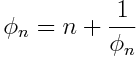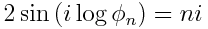# Imaginary gold, silver, bronze, …

The previous post gave a relationship between the imaginary unit i and the golden ratio. This post highlights a comment to that post explaining that the relationship generalizes to generalizations of the golden ratio.

GlennF pointed out that taking the larger root of the equationdefines the golden ratio when n = 1, the silver ratio when n = 2, etc. φn is also the continued fraction made entirely of n‘s.

With this definition, we have## 3 thoughts on “Imaginary gold, silver, bronze, …”

1. Andrew Stacey

Did you see the cosine version? Then you get 2 cos(i log(psi_n)) = n, where psi_n is a root of x + 1/x = n. (It’s too late in the evening for me to work out the relationship between psi_n and phi_n.)

2. The relation between \phi_n and \psi_m only seems to be a nice one for some cases. For example \psi_3 = (1 + \phi_1), which is what the cosine relation on Andrew Stacey’s page uses. There are others, e.g. \psi_6 = 1 + 2 \phi_2.

3. I would like a ration of gold better than golden ratio, I think. (Sorry, belatedly pointing out the typo.)